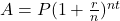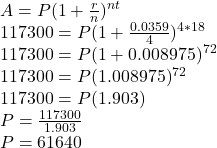Question

To help with her retirement savings, Donna has decided to invest. Assuming an interest rate of 3.59% compounded quarterly, how much would she have to invest to have $117,300 after 18 years? in progress 0 2 months 2021-08-01T17:23:48+00:00 1 Answers 3 views 0 ## Answers ( ) 1. Answer: We get the value of Principal amount i.e initial investment =$61640

Step-by-step explanation:

Interest rate r = 3.59% or 0.0359

Compounded quarterly n = 4

Future Amount A = 117,300

Time t = 18 years

We need to find initial investment i,e Principal Amount P

The formula used is:Putting values and finding PSo, We get the value of Principal amount i.e initial investment = \$61640